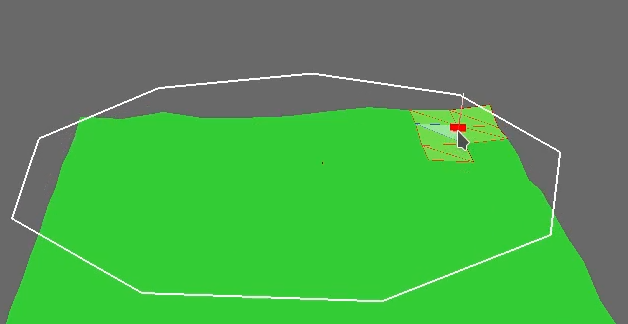# "Splash" texture onto mesh

I want to draw a texture dynamically onto a mesh (terrain mesh in my case), and wonder how that would be archived in panda3d.

In my case I want to to follow the shape I’m drawing above the terrain, projected down onto the terrain, but following it’s mesh shape. But for now I’m just wondering how to archive it in simple terms.

Here is my project so far:And something like this is what I want to achive (Forgive me my terrible painting skills.)The following code might not do exactly what you want (it doesn’t project shapes), but it might help with the interactive drawing (free-hand style, if I understood correctly):

``````from direct.showbase.ShowBase import ShowBase
from panda3d.core import *
import random
import array

base = ShowBase()

def create_terrain(x_size, y_size, max_height, resolution):

v_format = GeomVertexFormat.get_v3n3t2()
v_data = GeomVertexData("vertex_data", v_format, Geom.UH_static)
prim = GeomTriangles(Geom.UH_static)

mesh_data = array.array("f", [])
idx_data = array.array("H", [])
x_spacing = x_size / resolution
y_spacing = y_size / resolution

for i in range(resolution + 1):

x = x_spacing * i
u = i / resolution

for j in range(resolution + 1):
y = y_spacing * j
z = random.random() * max_height
v = j / resolution
mesh_data.extend([x, y, z, 0., 0., 1., u, v])

for i in range(resolution):
for j in range(resolution):
i1 = i * (resolution + 1) + j
i2 = i1 + resolution + 1
i3 = i2 + 1
i4 = i1 + 1
idx_data.extend([i1, i2, i3, i1, i3, i4])

vertex_count = (resolution + 1) * (resolution + 1)
v_data.unclean_set_num_rows(vertex_count)
view = memoryview(v_data.modify_array(0)).cast("B").cast("f")
view[:] = mesh_data

idx_array = prim.modify_vertices()
idx_array.unclean_set_num_rows(len(idx_data))
view = memoryview(idx_array).cast("B").cast("H")
view[:] = idx_data

geom = Geom(v_data)
node = GeomNode("terrain_node")
terrain_mesh = base.render.attach_new_node(node)
terrain_mesh.set_render_mode_filled_wireframe((1., 1., 1., 1.))
img = PNMImage(1, 1)
img.fill(0., 1., 0.)
tex = Texture()
terrain_mesh.set_texture(TextureStage.default, tex)

return terrain_mesh

# Setup camera

base.disable_mouse()
base.camera.set_hpr(10., -35, 0.)
base.camera.set_pos(10., -25., 25.)

# Setup a light source

p_light = PointLight("point_light")
base.light = base.camera.attach_new_node(p_light)
base.light.set_pos(5., -100., 7.)
base.render.set_light(base.light)

# Setup the terrain

terrain_x_size = 10.
terrain_y_size = 10.
terrain_mesh = create_terrain(terrain_x_size, terrain_y_size, 2., 6)
tex_size = 128
img = PNMImage(tex_size, tex_size, 4)  # has alpha channel for decal mode
tex = Texture()
ts = TextureStage("paint")
ts.sort = 1
ts.mode = TextureStage.M_decal
terrain_mesh.set_texture(ts, tex)

# Setup texture painting

brush_color = (0., 0., 1., 1.)
brush_size = 5
brush = PNMBrush.make_spot(brush_color, brush_size, True)
painter = PNMPainter(img)
painter.set_pen(brush)

# Create canvas plane to paint on

cm = CardMaker("canvas")
cm.set_frame(0., 1., 0., 1.)
canvas = base.render.attach_new_node(cm.generate())
canvas.set_p(-90.)
canvas.set_scale(terrain_x_size, 1., terrain_y_size)
canvas.set_pos(0., 0., 10.)
canvas.set_texture(ts, tex)
plane = Plane(Vec3.up(), Point3(0., 0., 10.))

if not base.mouseWatcherNode.is_button_down("mouse1"):

if not base.mouseWatcherNode.has_mouse():

mouse_pos = base.mouseWatcherNode.get_mouse()
world_pos = Point3()
near_point = Point3()
far_point = Point3()
base.camLens.extrude(mouse_pos, near_point, far_point)
near_point = base.render.get_relative_point(base.camera, near_point)
far_point = base.render.get_relative_point(base.camera, far_point)

if plane.intersects_line(world_pos, near_point, far_point):
canvas_pos = canvas.get_relative_point(base.render, world_pos)
x = canvas_pos.x * tex_size
y = (1. - canvas_pos.z) * tex_size
painter.draw_point(x, y)

base.run()
``````

When you run the code sample, you can press and hold the left mouse button to “paint” onto the flat plane that hovers above the (procedurally-generated) terrain mesh (you should of course load your own terrain model).
The same texture is applied to both the plane and the terrain to make it easier to see how the painting follows the mouse cursor. It is applied in “decal” mode to preserve the color of the “brush” (in “modulate” mode it would get multiplied with the underlying color).

If there’s anything that requires clarification, don’t hesitate to ask!

At any rate, I hope it will prove helpful to some extent, at least.1 Like

Looks good, although not quite what I was trying to accomplish, I think I might be able to create a solution from the code you posted.

I’m trying to do something like this ():This can be done using texturing modes.
https://docs.panda3d.org/1.10/python/programming/texturing/texture-modes#blend-mode

or:

https://docs.panda3d.org/1.10/python/programming/texturing/projected-textures#id2

3 Likes

Ah, looks like I misunderstood, then!

Indeed, texture projection seems like a good solution.
An alternative could be to just use texture transformations.